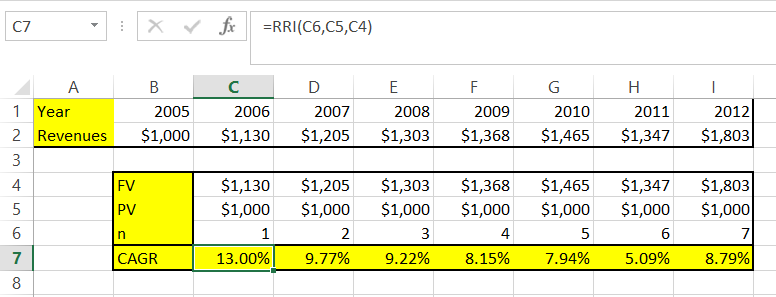## How To Calculate Cagr?

In this lesson you can learn how to calculate CAGR in Excel.

CAGR (Compound Annual Growth Rate) is year-of-year average growth rate over a period of time. Calculating CAGR you can check how much do you earn annually with your investments.

There are two ways to calculate CAGR in Excel.

Method 1. To calculate CAGR you can use that formula:

= ((FV/PV)^(1/n)) – 1

• FV stands for Future Value
• PV stands for Present Value
• n stands for count of investment yearsPicture show the example of formula. I think everything is clear.

Method 2. The other way is to use RRI Excel function.

RRI function takes 3 arguments:

• nper (the same as n in the previous example)
• PV
• FV

In such situation the function in given example will be:

=RRI(C6,C5,C4)The results are the same so it proves that both of the methods are working the same way.

## Template

```Further reading: